# Performance of Sparse Recovery Using L1 Minimization¶

Important: Please read the installation page for details about how to install the toolboxes. $\newcommand{\dotp}{\langle #1, #2 \rangle}$ $\newcommand{\enscond}{\lbrace #1, #2 \rbrace}$ $\newcommand{\pd}{ \frac{ \partial #1}{\partial #2} }$ $\newcommand{\umin}{\underset{#1}{\min}\;}$ $\newcommand{\umax}{\underset{#1}{\max}\;}$ $\newcommand{\umin}{\underset{#1}{\min}\;}$ $\newcommand{\uargmin}{\underset{#1}{argmin}\;}$ $\newcommand{\norm}{\|#1\|}$ $\newcommand{\abs}{\left|#1\right|}$ $\newcommand{\choice}{ \left\{ \begin{array}{l} #1 \end{array} \right. }$ $\newcommand{\pa}{\left(#1\right)}$ $\newcommand{\diag}{{diag}\left( #1 \right)}$ $\newcommand{\qandq}{\quad\text{and}\quad}$ $\newcommand{\qwhereq}{\quad\text{where}\quad}$ $\newcommand{\qifq}{ \quad \text{if} \quad }$ $\newcommand{\qarrq}{ \quad \Longrightarrow \quad }$ $\newcommand{\ZZ}{\mathbb{Z}}$ $\newcommand{\CC}{\mathbb{C}}$ $\newcommand{\RR}{\mathbb{R}}$ $\newcommand{\EE}{\mathbb{E}}$ $\newcommand{\Zz}{\mathcal{Z}}$ $\newcommand{\Ww}{\mathcal{W}}$ $\newcommand{\Vv}{\mathcal{V}}$ $\newcommand{\Nn}{\mathcal{N}}$ $\newcommand{\NN}{\mathcal{N}}$ $\newcommand{\Hh}{\mathcal{H}}$ $\newcommand{\Bb}{\mathcal{B}}$ $\newcommand{\Ee}{\mathcal{E}}$ $\newcommand{\Cc}{\mathcal{C}}$ $\newcommand{\Gg}{\mathcal{G}}$ $\newcommand{\Ss}{\mathcal{S}}$ $\newcommand{\Pp}{\mathcal{P}}$ $\newcommand{\Ff}{\mathcal{F}}$ $\newcommand{\Xx}{\mathcal{X}}$ $\newcommand{\Mm}{\mathcal{M}}$ $\newcommand{\Ii}{\mathcal{I}}$ $\newcommand{\Dd}{\mathcal{D}}$ $\newcommand{\Ll}{\mathcal{L}}$ $\newcommand{\Tt}{\mathcal{T}}$ $\newcommand{\si}{\sigma}$ $\newcommand{\al}{\alpha}$ $\newcommand{\la}{\lambda}$ $\newcommand{\ga}{\gamma}$ $\newcommand{\Ga}{\Gamma}$ $\newcommand{\La}{\Lambda}$ $\newcommand{\si}{\sigma}$ $\newcommand{\Si}{\Sigma}$ $\newcommand{\be}{\beta}$ $\newcommand{\de}{\delta}$ $\newcommand{\De}{\Delta}$ $\newcommand{\phi}{\varphi}$ $\newcommand{\th}{\theta}$ $\newcommand{\om}{\omega}$ $\newcommand{\Om}{\Omega}$

This tour explores theoritical garantees for the performance of recovery using $\ell^1$ minimization.

In :
addpath('toolbox_signal')


## Sparse $\ell^1$ Recovery¶

We consider the inverse problem of estimating an unknown signal $x_0 \in \RR^N$ from noisy measurements $y=\Phi x_0 + w \in \RR^P$ where $\Phi \in \RR^{P \times N}$ is a measurement matrix with $P \leq N$, and $w$ is some noise.

This tour is focused on recovery using $\ell^1$ minimization $$x^\star \in \uargmin{x \in \RR^N} \frac{1}{2}\norm{y-\Phi x}^2 + \la \normu{x}.$$

Where there is no noise, we consider the problem $\Pp(y)$ $$x^\star \in \uargmin{\Phi x = y} \normu{x}.$$

We are not concerned here about the actual way to solve this convex problem (see the other numerical tours on sparse regularization) but rather on the theoritical analysis of wether $x^\star$ is close to $x_0$.

More precisely, we consider the following three key properties

• Noiseless identifiability: $x_0$ is the unique solution of $\Pp(y)$ for $y=\Phi x_0$.
• Robustess to small noise:: one has $\norm{x^\star - x_0} = O(\norm{w})$ for $y=\Phi x_0+w$ if $\norm{w}$ is smaller than an arbitrary small constant that depends on $x_0$ if $\la$ is well chosen according to $\norm{w}$.
• Robustess to bounded noise: same as above, but $\norm{w}$ can be arbitrary.

Note that noise robustness implies identifiability, but the converse is not true in general.

## Coherence Criteria¶

The simplest criteria for identifiality are based on the coherence of the matrix $\Phi$ and depends only on the sparsity $\norm{x_0}_0$ of the original signal. This criteria is thus not very precise and gives very pessimistic bounds.

The coherence of the matrix $\Phi = ( \phi_i )_{i=1}^N \in \RR^{P \times N}$ with unit norm colum $\norm{\phi_i}=1$ is $$\mu(\Phi) = \umax{i \neq j} \abs{\dotp{\phi_i}{\phi_j}}.$$

Compute the correlation matrix (remove the diagonal of 1's).

In :
remove_diag = @(C)C-diag(diag(C));
Correlation = @(Phi)remove_diag(abs(Phi'*Phi));


Compute the coherence $\mu(\Phi)$.

In :
maxall = @(C)max(C(:));
mu = @(Phi)maxall(Correlation(Phi));


The condition $$\normz{x_0} < \frac{1}{2}\pa{1 + \frac{1}{\mu(\Phi)}}$$ implies that $x_0$ is identifiable, and also implies to robustess to small and bounded noise.

Equivalently, this condition can be written as $\text{Coh}(\normz{x_0})<1$ where $$\text{Coh}(k) = \frac{k \mu(\Phi)}{ 1 - (k-1)\mu(\Phi) }$$

In :
Coh = @(Phi,k)(k * mu(Phi)) / ( 1 - (k-1) * mu(Phi) );


Generate a matrix with random unit columns in $\RR^P$.

In :
normalize = @(Phi) Phi ./ repmat(sqrt(sum(Phi.^2)), [size(Phi,1) 1]);
PhiRand = @(P,N)normalize(randn(P,N));
Phi = PhiRand(250,1000);


Compute the coherence and the maximum possible sparsity to ensure recovery using the coherence bound.

In :
c = mu(Phi);
fprintf('Coherence:    %.2f\n', c);
fprintf('Sparsity max: %d\n', floor(1/2*(1+1/c)) );

Coherence:    0.30
Sparsity max: 2


Exercise 1

Display how the average coherence of a random matrix decays with the redundancy $\eta = P/N$ of the matrix $\Phi$. Can you derive an empirical law between $P$ and the maximal sparsity? = plot(eta_list, k_mean ); set(h, 'LineWidth', 2); abel('\eta'); ylabel('1/2(1+1/E(\mu))');

In :
exo1()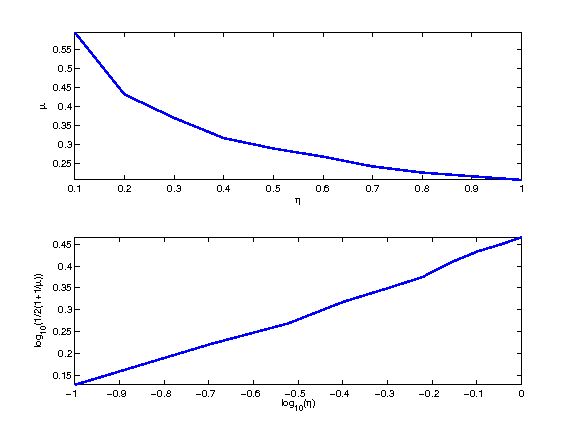In :
%% Insert your code here.


## Support and Sign-based Criteria¶

In the following we will consider the support $$\text{supp}(x_0) = \enscond{i}{x_0(i) \neq 0}$$ of the vector $x_0$. The co-support is its complementary $I^c$.

In :
supp   = @(s)find(abs(s)>1e-5);
cosupp = @(s)find(abs(s)<1e-5);


Given some support $I \subset \{0,\ldots,N-1\}$, we will denote as $\Phi = (\phi_m)_{m \in I} \in \RR^{N \times \abs{I}}$ the sub-matrix extracted from $\Phi$ using the columns indexed by $I$.

J.J. Fuchs introduces a criteria $F$ for identifiability that depends on the sign of $x_0$.

J.J. Fuchs. Recovery of exact sparse representations in the presence of bounded noise. IEEE Trans. Inform. Theory, 51(10), p. 3601-3608, 2005

Under the condition that $\Phi_I$ has full rank, the $F$ measure of a sign vector $s \in \{+1,0,-1\}^N$ with $\text{supp}(s)=I$ reads $$\text{F}(s) = \norm{ \Psi_I s_I }_\infty \qwhereq \Psi_I = \Phi_{I^c}^* \Phi_I^{+,*}$$ where $A^+ = (A^* A)^{-1} A^*$ is the pseudo inverse of a matrix $A$.

The condition $$\text{F}(\text{sign}(x_0))<1$$ implies that $x_0$ is identifiable, and also implies to robustess to small noise. It does not however imply robustess to a bounded noise.

Compute $\Psi_I$ matrix.

In :
PsiI = @(Phi,I)Phi(:, setdiff(1:size(Phi,2),I) )' * pinv(Phi(:,I))';


Compute $\text{F}(s)$.

In :
F = @(Phi,s)norm(PsiI(Phi,supp(s))*s(supp(s)), 'inf');


The Exact Recovery Criterion (ERC) of a support $I$, introduced by Tropp in

J. A. Tropp. Just relax: Convex programming methods for identifying sparse signals. IEEE Trans. Inform. Theory, vol. 52, num. 3, pp. 1030-1051, Mar. 2006.

Under the condition that $\Phi_I$ has full rank, this condition reads $$\text{ERC}(I) = \norm{\Psi_{I}}_{\infty,\infty} = \umax{j \in I^c} \norm{ \Phi_I^+ \phi_j }_1.$$ where $\norm{A}_{\infty,\infty}$ is the $\ell^\infty-\ell^\infty$ operator norm of a matrix $A$, computed with the Matlab command |norm(A,'inf')|.

In :
erc = @(Phi,I)norm(PsiI(Phi,I), 'inf');


The condition $$\text{ERC}(\text{supp}(x_0))<1$$ implies that $x_0$ is identifiable, and also implies to robustess to small and bounded noise.

One can prove that the ERC is the maximum of the F criterion for all signs of the given support $$\text{ERC}(I) = \umax{ s, \text{supp}(s) \subset I } \text{F}(s).$$

The weak-ERC is an approximation of the ERC using only the correlation matrix $$\text{w-ERC}(I) = \frac{ \umax{j \in I^c} \sum_{i \in I} \abs{\dotp{\phi_i}{\phi_j}} }{ 1-\umax{j \in I} \sum_{i \neq j \in I} \abs{\dotp{\phi_i}{\phi_j}} }$$

In :
g = @(C,I)sum(C(:,I),2);
werc_g = @(g,I,J)max(g(J)) / (1-max(g(I)));
werc = @(Phi,I)werc_g( g(Correlation(Phi),I), I, setdiff(1:size(Phi,2),I) );


One has, if $\text{w-ERC}(I)>0$, for $I = \text{supp}(s)$, $$\text{F}(s) \leq \text{ERC}(I) \leq \text{w-ERC}(I) \leq \text{Coh}(\abs{I}).$$

This shows in particular that the condition $$\text{w-ERC}(\text{supp}(x_0))<1$$ implies identifiability and robustess to small and bounded noise.

Exercise 2

Show that this inequality holds on a given matrix. What can you conclude about the sharpness of these criteria ?

In :
exo2()

N=2000, P=1990, |I|=6
F(s)    =0.19
ERC(I)  =0.25
w-ERC(s)=0.27
Coh(|s|)=1.78

In :
%% Insert your code here.


Exercise 3

For a given matrix $\Phi$ generated using |PhiRand|, draw as a function of the sparsity $k$ the probability that a random sign vector $s$ of sparsity $\norm{s}_0=k$ satisfies the conditions $\text{F}(x_0)<1$, $\text{ERC}(x_0)<1$ and $\text{w-ERC}(x_0)<1$

In :
exo3()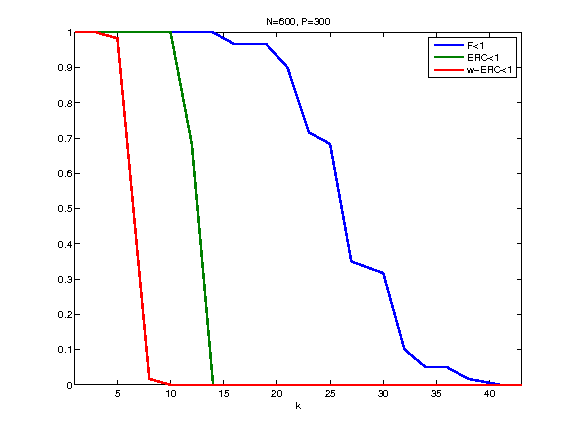In :
%% Insert your code here.


## Restricted Isometry Criteria¶

The restricted isometry constants $\de_k^1,\de_k^2$ of a matrix $\Phi$ are the smallest $\de^1,\de^2$ that satisfy $$\forall x \in \RR^N, \quad \norm{x}_0 \leq k \qarrq (1-\de^1)\norm{x}^2 \leq \norm{\Phi x}^2 \leq (1+\de^2)\norm{x}^2.$$

E. Candes shows in

E. J. Cand s. The restricted isometry property and its implications for compressed sensing. Compte Rendus de l'Academie des Sciences, Paris, Serie I, 346 589-592

that if $$\de_{2k} \leq \sqrt{2}-1 ,$$ then $\norm{x_0} \leq k$ implies identifiability as well as robustness to small and bounded noise.

The stability constant $\la^1(A), \la^2(A)$ of a matrix $A = \Phi_I$ extracted from $\Phi$ is the smallest $\tilde \la_1,\tilde \la_2$ such that $$\forall \al \in \RR^{\abs{I}}, \quad (1-\tilde\la_1)\norm{\al}^2 \leq \norm{A \al}^2 \leq (1+\tilde \la_2)\norm{\al}^2.$$

These constants $\la^1(A), \la^2(A)$ are easily computed from the largest and smallest eigenvalues of $A^* A \in \RR^{\abs{I} \times \abs{I}}$

In :
minmax = @(v)deal(1-min(v),max(v)-1);
ric = @(A)minmax(eig(A'*A));


The restricted isometry constant of $\Phi$ are computed as the largest stability constants of extracted matrices $$\de^\ell_k = \umax{ \abs{I}=k } \la^\ell( \Phi_I ).$$

The eigenvalues of $\Phi$ are essentially contained in the interval $[a,b]$ where $a=(1-\sqrt{\be})^2$ and $b=(1+\sqrt{\be})^2$ with $\beta = k/P$ More precisely, as $k=\be P$ tends to infinity, the distribution of the eigenvalues tends to the Marcenko-Pastur law $f_\be(\la) = \frac{1}{2\pi \be \la}\sqrt{ (\la-b)^+ (a-\la)^+ }.$

Exercise 4

Display, for an increasing value of $k$ the histogram of repartition of the eigenvalues $A^* A$ where $A$ is a Gaussian matrix of size $(P,k)$ and variance $1/P$. For this, accumulate the eigenvalues for many realization of $A$.

In :
exo4()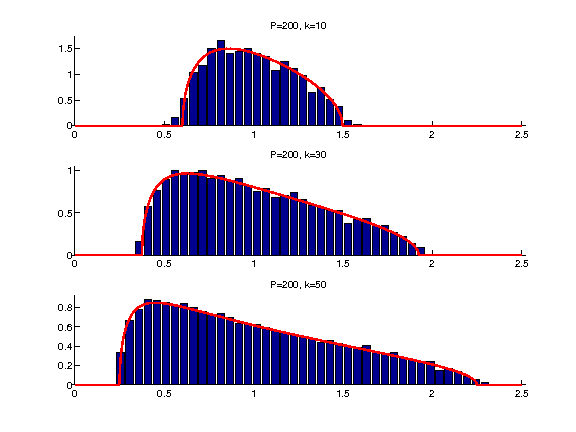In :
%% Insert your code here.


Exercise 5

Estimate numerically lower bound on $\de_k^1,\de_k^2$ by Monte-Carlo sampling of sub-matrices.

In :
exo5()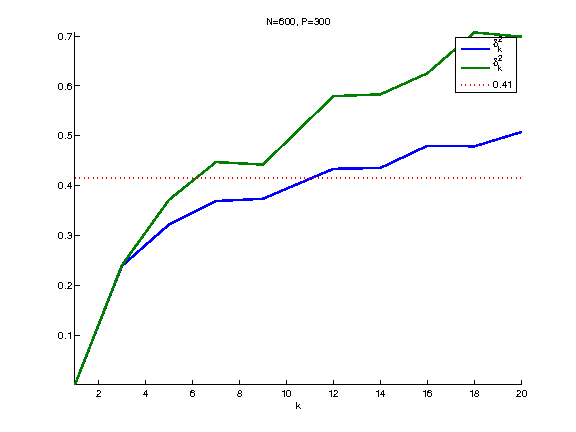In :
%% Insert your code here.


## Sparse Spikes Deconvolution¶

We now consider a convolution dictionary $\Phi$. Such a dictionary is used with sparse regulariz

Second derivative of Gaussian kernel $g$ with a given variance $\si^2$.

In :
sigma = 6;
g = @(x)(1-x.^2/sigma^2).*exp(-x.^2/(2*sigma^2));


Create a matrix $\Phi$ so that $\Phi x = g \star x$ with periodic boundary conditions.

In :
P = 1024;
[Y,X] = meshgrid(1:P,1:P);
Phi = normalize(g(mod(X-Y+P/2, P)-P/2));


To improve the conditionning of the dictionary, we sub-sample its atoms, so that $P = \eta N > N$.

In :
eta = 2;
N = P/eta;
Phi = Phi(:,1:eta:end);


Plot the correlation function associated to the filter. Can you determine the value of the coherence $\mu(\Phi)$?

In :
c = Phi'*Phi;
c = abs(c(:,end/2));
clf;
h = plot(c(end/2-50:end/2+50), '.-'); set(h, 'LineWidth', 1);
axis tight;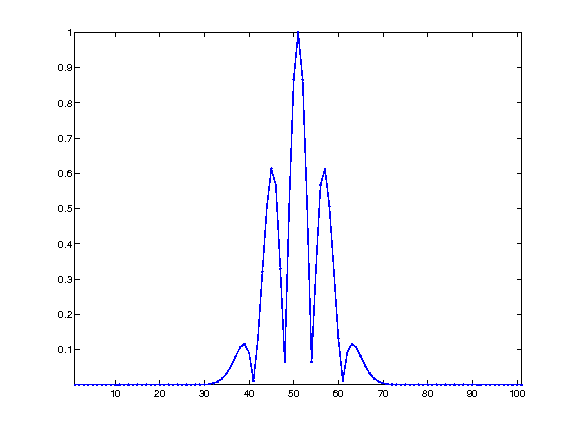Create a data a sparse $x_0$ with two diracs of opposite signes with spacing $d$.

In :
twosparse = @(d)circshift([1; zeros(d,1); -1; zeros(N-d-2,1)], round(N/2-d/2));


Display $x_0$ and $\Phi x_0$.

In :
x0 = twosparse(50);
clf;
subplot(2,1,1);
h = plot(x0, 'r'); axis tight;
subplot(2,1,2);
h = plot(Phi*x0, 'b'); axis tight;
set(h, 'LineWidth', 2);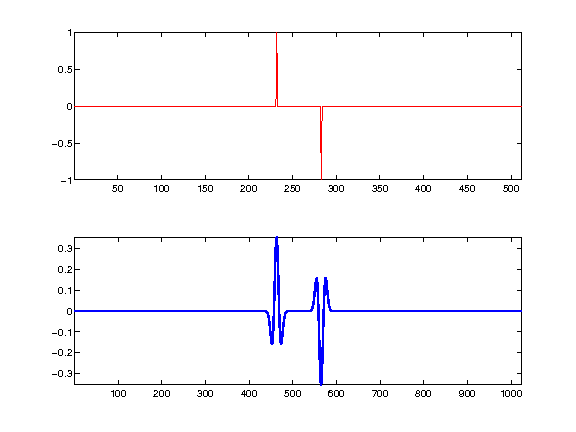Exercise 6

Plot the evolution of the criteria F, ERC and Coh as a function of $d$. Do the same plot for other signs patterns for $x_0$. Do the same plot for a Dirac comb with a varying spacing $d$.

In :
exo6()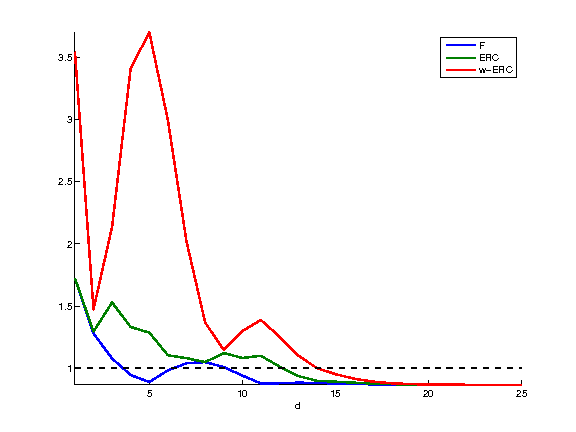In :
%% Insert your code here.